# Day 1: Ionic Compound Formulas

16 teachers like this lesson
Print Lesson

## Objective

SWBAT determine molecular formulas for ionic compounds using a periodic table and a list of polyatomic ions.

#### Big Idea

Ionic compounds have an overall neutral charge which determines their molecular formulas.

## Why This Lesson?

This lesson helps students understand that the molecular formula for an ionic compound is electrically neutral and can be determined by finding the ratio of cations to anions that results in an overall zero charge.  Students need to understand this concept as a precursor to studying ionic chemical reactions and acid-base chemistry.

This lesson addresses the NGSS Performance Expectations of HS-PS1-1 and HS-PS1-2 because students are using position on the periodic table to determine ionic charges (PS1-1) and then will be using their understanding of ionic compounds to predict results of chemical reactions (PS1-2).  Students have already investigated dissolution of ionic compounds and know that the ions in solution conduct electricity.  Later, we will investigate the strength of acids and bases based on level of conductivity, because strong acids/bases dissociate fully into ions.  A basic understanding of ionic compounds and their dissociation will help students understand acids and bases later.

Students will be using simple paper models to demonstrate charge ratio (SP 2) and then explain their reasoning for their determined molecular formula of the assigned ionic compounds (SP 6), addressing two of the Science and Engineering Practices.

## Warm-Up

5 minutes

While I take attendance, students do a warm-up activity in their composition Warm-Up/Reflection books.  I use warm-ups to either probe for students' prior knowledge about the day's upcoming lesson or to have them bring to mind and review what they should have learned previously.  (To read more about Warm Up and Reflection Books, please see the attached resource.)

Today's Warm-Up: "How do we know what the ionization charge (the number of electrons an element will gain or lose and the resulting charge) will be for particular elements?"

In this case, the warm-up is asking students to recall the previous day's lesson in which they worked to determine ionization charges of various elements.  It is also preparing students to apply that learning to today's activity in which they determine ionic compound formulas.

If time permits, I walk around with a self-inking stamp to stamp the completed warm-ups indicating participation, but not necessarily accuracy.  On days when there is too much business keeping, I do not stamp.  Students have been told that warm-ups are occasionally immediately checked and other times not.  At the end of each unit, Warm-Up/Reflection Books are collected and spot-checked.

## Intro to Activity

10 minutes

In the previous day's lesson, we focused on how to determine whether an element tends to gain or lose electrons, and how many electrons are involved.  At this point, students should have an understanding that the periodic table is set up in such a way that we can use an element's position to determine its most likely ionic state.  Using this knowledge, students should be able to predict charges for alkali metals, alkaline earth metals, aluminum, halogens, and oxygen group nonmetals.

I pass out the handouts entitled Ionic Bonds so that each student has one.  At the whiteboard, I remind students how to determine what ions are formed by each element as predicted by the periodic table.  I compare the elements' total number of electrons to the nearest noble gas' number of electrons to reinforce the idea that elements only gain or lose electrons to be more stable, which the noble gases already are and the other elements want to be.

I model how the first line in the table would be figured out and explain that the cations are listed first (and remind students that cations are positive) while the anions are listed second (also reminding that anions are negative).  I explain that the "# cations" and "# anions" titles are not hashtags (!) and that they are also not the charges, but rather the number of ions necessary to create an electrically neutral compound.

Using MgCl as the example, I explain in this way:

## Partner Activity

30 minutes

Students work in pairs that are pre-determined by their Partners Sheet (see the Reflection entitled "Compass Pairs" in my Common Ionization States lesson).  I tell students that one person of the pair is responsible for preparing the anions and the other is responsible for the cations, and I pass out the ANIONS sheet and CATIONS sheet to each group.  I have previously set out scissors at the student tables and I tell students to cut along the bold lines of the anion and cation sheets to make the individual ions.  I explain that the pieces with  “+” correspond to an ion with +1 charge, the “+ +” pieces correspond to ions with a +2 charge, and the “+ + +” pieces correspond to ions with a +3 charge.  Likewise for the anions (one “-“ equals -1 charge, etc.).

I have designed the pieces so that their size corresponds to how many positive or negative charges it carries, so that when students are trying to balance charges, they can do it by matching the sizes of the charge papers.  Once they have balanced the charges in a compound, they can count up cations and anions to determine what the subscripts are in the chemical formula by following the scaffolded steps in the table on the handout.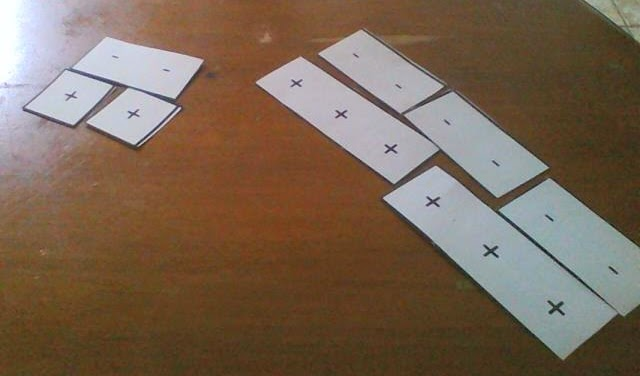Two different compounds are pictured here.  The one on the left is composed of cations charged +1 and anions charged -2.  By matching charges until all charges are balanced between positive and negative, the students can see that there are 2 cations needed and 1 anion, so the chemical formula would be Cation(subscript=2) Anion(subscript=1).  Likewise, the compound on the right is composed of cations with charge +3 and anions with charge -2.  Here we see that 2 cations and 3 anions are needed for all 6 positive and negative charges to be balanced which means the chemical formula would be Cation(subscript=2) Anion(subscript=3).

A key for the handout can be found here: Ionic Bonds KEY

I developed the charges model once I noticed that my students were not understanding that the subscripts indicate how many of each ion are necessary to make a neutral ionic compound.  Here are some samples of student work that were the driving force behind revisiting how I teach ionic compound formulas: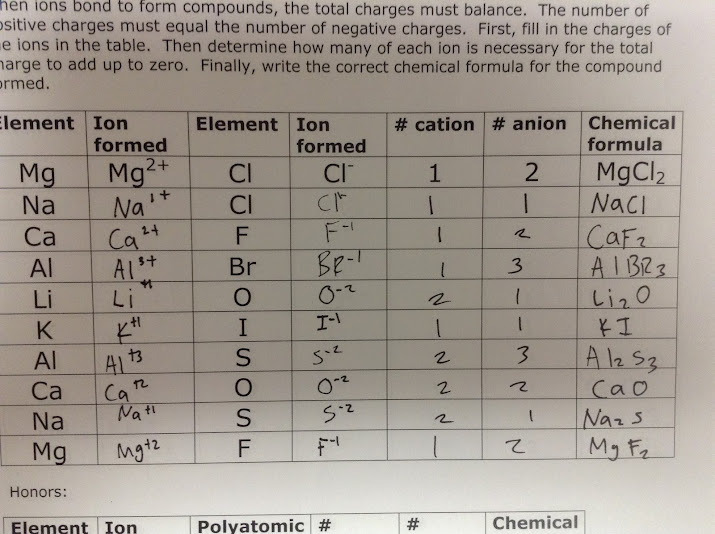Both the student above and the student below misunderstood how the quantities of each ion relate to helping to balance the charges.  In particular, CaO seemed to be an inordinately difficult chemical formula to generate correctly for most students.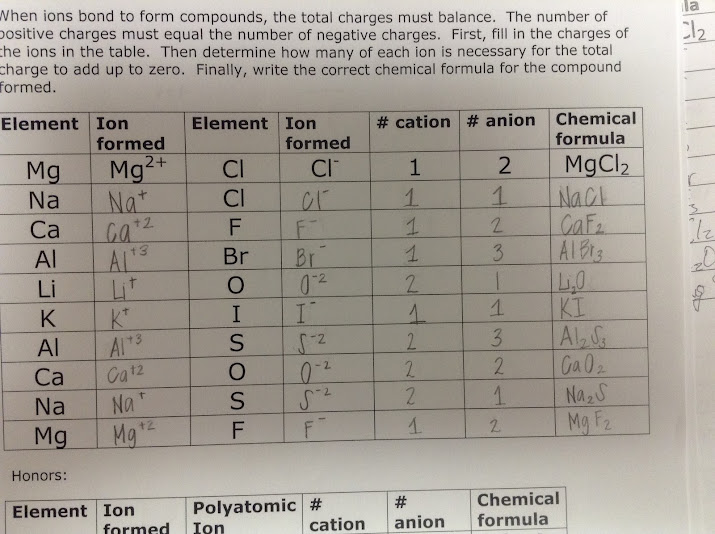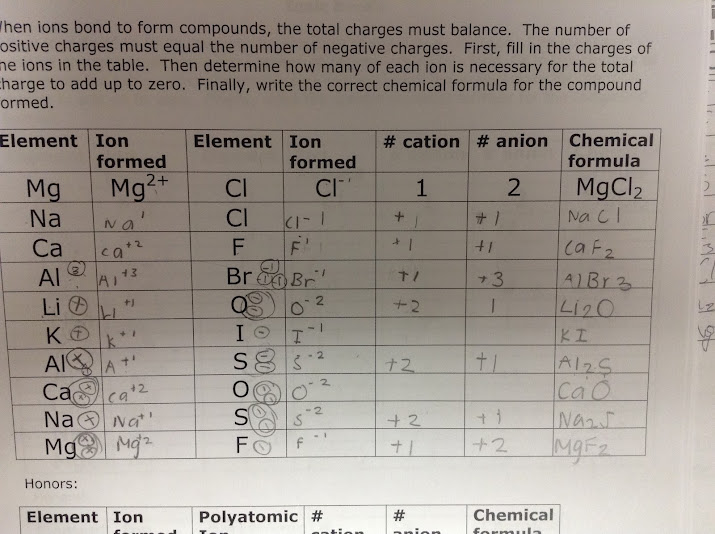For both the above sample and the below sample, students began writing charges (with my suggestion) to try to arrive at neutral chemical formulas.  When this started to work for several struggling students, I thought making some type of manipulative for students to use as they work through the activity would be helpful.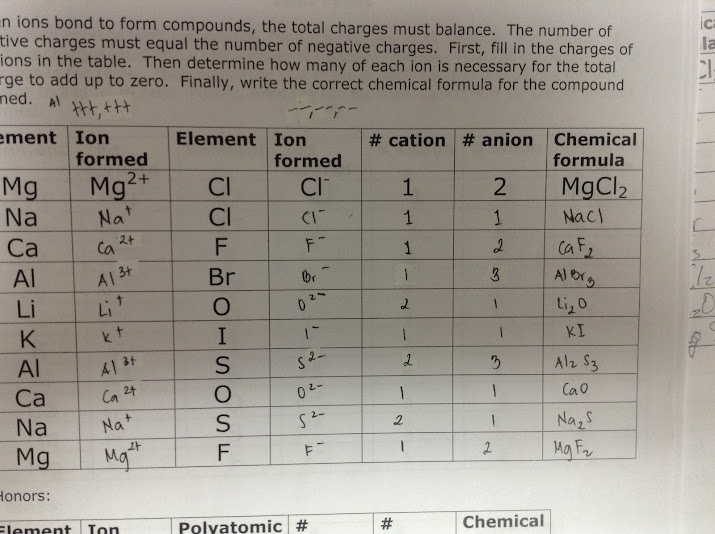## Student Reflection

5 minutes

In student's Warm-Up/Reflection Books, students should spend about 3-5 minutes writing a response to the day's reflection prompt.  Prompts are designed to either help students focus on key learning goals from the day's lesson or to prompt deeper thinking.  The responses also allow me to see if there are any students who are missing the mark in terms of understanding.  The collection of responses in the composition books can also show a progression (or lack thereof) for individual students.

Today's Reflection Prompt:  "How do we find ionic compound chemical formulas?"

Desired student responses should include:

• We need to find the ionization charges for both the anions and cations in the compound
• The chemical formula must be neutral, meaning the total number of positive charges equals the total number of negative charges
• We can change the amounts of each ion we have until the positives equal the negatives.  We write these amounts (numbers of each ion) as subscripts in the chemical formula.